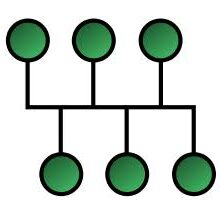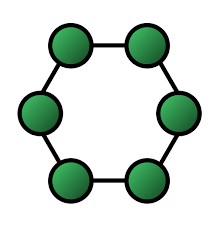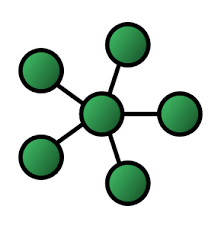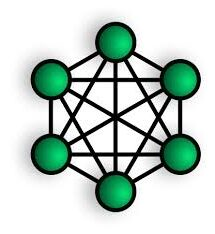# Network Topology – Meaning and Types Network Topology Primary 6 (Basic 6) Term 2 Week 5 Information Technology

### COMPUTER STUDIES

BASIC SCIENCE AND TECHNOLOGY

SECOND TERM

WEEK 5

PRIMARY 6

THEME – BASIC COMPUTER OPERATION

PREVIOUS LESSON – Local Area Network, Metropolitan Area Network and Large or Wide Area Network Primary 6 (Basic 6) Term 2 Week 4 Information Technology

### LEARNING AREA

1. Introduction

2. Meaning of Network Topology

3. Types of Network Topology

4. Stretch Different Types of Network Topology

### PERFORMANCE OBJECTIVES

By the end of the lesson, most of the pupils should have attained the following objectives –

1. describe network topology.

2. state types of network topology

3. sketch and describe bus, ring and star topology.

### ENTRY BEHAVIOR

The pupils can state the requirements for computer network.

### INSTRUCTIONAL MATERIALS

The teacher will teach the lesson with the aid of charts of network topology.

### METHOD OF TEACHING

Choose a suitable and appropriate methods for the lessons.

Note – Irrespective of choosing methods of teaching, always introduce an activities that will arouse pupil’s interest or lead them to the lessons.

### REFERENCE MATERIALS

Scheme of Work

9 – Years Basic Education Curriculum

Course Book – Tanus Computer Studies book 6

All Relevant Material

Online Information

### LESSON 1 – INTRODUCTION

MEANING OF NETWORK TOPOLOGY

Network topology is the arrangement and description of a computer network.

TYPES OF NETWORK TOPOLOGY

The types of computer network are as follows:

1. Bus topology

2. Ring topology

3. Star topology

1. BUS TOPOLOGY

Bus topology is a type of network where all the computers are connected to a single cable.2. RING TOPOLOGY

Ring topology is a type of network where  all computer are connected to create a circular data path.3. STAR TOPOLOGY

Star topology is a network where all computer are connected at a central point.3. MESH TOPOLOGY

Mesh topology is a network where all the computer are interconnected with one another.5. TREE TOPOLOGY

Tree topology is a network where all the computers are connected like a branches of a tree.6. POINT TO POINT TOPOLOGY

Point to point topology is a network where two or more computer are connected to one another direct in a line.LESSON 2 – SKETCHING OF BUS AND RING TOPOLOGY

Teacher organizes pupils in groups or pairs depending on the size of the class to sketch and describe the above computer network on cardboard.

LESSON 3 – REVISION AND WEEKLY ASSESSMENT (TEST)

### PRESENTATION

To deliver the lesson, the teacher adopts the following steps:

1. To introduce the lesson, the teacher revises the previous lesson. Based on this, he/she asks the pupils some questions;

2. Organizes the pupils in groups or pair depending on the size of the class.

3. Displays charts showing types of network topology.

4. Lets pupils different each of the network based on the arrangement.

Pupil’s Activities – Identify the setup of each network topology.

5. Uses the chart to introduce and explain each type of topology.

Pupil’s Activities – Listen to the teacher’s explanation.

6. Gets two or more functional computers to illustration each topology.

Pupil’s Activities – Support the teacher in setup each network topology.

7. Sketch and ask pupils to sketch each network topology.

Pupil’s Activities – Sketch all the network topology.

8. Summarizes the lessons on the board with appropriate lesson evaluation.

Pupil’s Activities – Participate act actively in the summary of the lesson by responding correctly to questions and write as instructed.

### CONCLUSION

• To conclude the lesson for the week, the teacher revises the entire lesson and links it to the following week’s lesson.

### LESSON EVALUATION

1. explain the meaning of network topology.

2. list and explain any 5 network topology with appropriate drawing.

### WEEKLY ASSESSMENT

1. ________ is the arrangement and description of a computer network.

A. Computer network

B. Computer system

C. Computer network topology

D. Computer hardware

2. There are ________ types of network topology.

A. 3

B. 4

C. 5

D. 6

3. ________ types of network topology looks like a circle.

A. Bus topology

B. Ring topology

C. Star topology

D. Circle topology

4. ________ types of network topology looks like a sitting bus.

A. Bus topology

B. Ring topology

C. Star topology

D. Mesh topology

5. ________ types of network topology looks like a star.

A. Bus topology

B. Ring topology

C. Star topology

D. Circle topology

6. Network topology is the ________ and ________ of a computer network.

7. ________ is a type of network where all the computers are connected to a single cable.

A. Point to point topology

B. Ring topology

C. Bus topology

D. Mesh topology

8. ________ is a type of network where  all computer are connected to create a circular data path.

A. Bus topology

B. Ring topology

C. Star topology

D. Mesh topology

9. ________ is a network where all computer are connected at a central point.

A. Bus topology

B. Star topology

C. Tree topology

D. Ring topology

10. ________ is a network where all the computer are interconnected with one another.

A. Mesh topology

B. Ring topology

C. Star topology

D. Bus topology

11. ________ is a network where all the computers are connected like a branches of a tree.

A. Point to point topology

B. Ring topology

C. Tree topology

D. Mesh topology

12. ________ is a network where two or more computer are connected to one another direct in a line.

A. Point to point topology

B. Ring topology

C. Bus topology

D. Mesh topology

13. Bus topology is a network where two or more computer are connected to one another direct in a line.

A. True

B. False

14. Star topology is a network where all the computers are connected like a branches of a tree.

A. False

B. True

15. Mesh topology is a network where all the computer are interconnected with one another.

A. False

B. True

16. Bus topology is a type of network where all the computers are connected to a single cable.

A. False

B. True

17. Sketch a mesh topology.

18. Sketch a star topology.

19. Sketch a bus topology.

20. Sketch a tree topology.